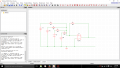# Op Amp circuit design for required Vout

#### CNC682

Joined Jan 23, 2015
27
Q. the block diagram of an electronic system required to
give the output Vo = 8(V4) + 4(V3) + 2(V2) + V1.

(a)
Sketch a suitable op-amp circuit to give the required output and show
the required resistor ratios.

Vo=8(V4)+4(V3)+2(V2)+V1. I need to design an Op Amp circuit and provide the required resistor ratio values using the "Vo" equation. I know it is a summing amplifier circuit required and the amplification factor, in case of V4 for example is 8. I know that amplification factor is equal to -Rf(feedback resistor) divided by R1,R2 etc (-Rf/R.) If i can find Rf then the rest is easy, any ideas?

This my design. Rf being R5 in the picture below#### bertus

Joined Apr 5, 2008
22,133
Hello,

All resistors in your schematic have the value 0.
This will not work.
Also are the powerconnections of the opamp made?

Bertus

#### CNC682

Joined Jan 23, 2015
27
The schematic is only a digital sketch if you like, i need to calculate the resistor values from the required output- Vo=8(V4)+4(V3)+2(V2)+V1. If i can calculate the feedback resistor then the other resistor values will be easy to calculate

#### Jony130

Joined Feb 17, 2009
5,439
First assume that Rf resistor has a given value equal to Rf, next find all other resistors.

#### dannyf

Joined Sep 13, 2015
2,197
First you need negative feedback for it to work.

Once you have that resolved, you need to calculate the current flowing through the resistors and that will provide you with the right resistor value.

#### CNC682

Joined Jan 23, 2015
27
Ok, If I assume that Rf is 100k and Amplification factor=Rf/Rn then for the remaining resistor values we have:

For V4: 8=100k/R4 . R4= 12.5k
For V3: 4=100k/R3 . R3=25k
For V2: 2=100k/R2 . R2=50k
For V1: 1=100k/R1 . R1=100k

Can I really just assume Rf?

#### JoeJester

Joined Apr 26, 2005
4,390
Sure you can assume Rf. Then you might only have E24 series resistors on hand and you will have to ensure your choice is the closest to your design constraints.

#### Attachments

• 28.6 KB Views: 11

#### CNC682

Joined Jan 23, 2015
27
First you need negative feedback for it to work.

Once you have that resolved, you need to calculate the current flowing through the resistors and that will provide you with the right resistor value.
How do I calculate current without voltage values?

#### shteii01

Joined Feb 19, 2010
4,644
You are not assuming value of Rf. You are CHOOSING value of Rf. You are DESIGNING a circuit, YOU, THE DESIGNER, CHOOSE what goes into the circuit.

1) Your Rf, R5 in your circuit, is in the wrong place.
2) You are supposed to have two feedback resistors.
3) Gain of non-inverting summing amplifier is $$1+\frac{Rf2}{Rf1}$$

Examine this material for better explanation and example:
http://microchip.wikidot.com/asp0107:non-inverting-summing-amp

http://masteringelectronicsdesign.c...f-the-summing-amplifier-with-n-input-signals/

Last edited:

#### CNC682

Joined Jan 23, 2015
27
Apologies just realised my "sketch" has the op amp upside down. It should be an inverting amplifier, therefore I only need one feedback resistor and it is in the right place if I look at inverting examples.

#### shteii01

Joined Feb 19, 2010
4,644
Apologies just realised my "sketch" has the op amp upside down. It should be an inverting amplifier, therefore I only need one feedback resistor and it is in the right place if I look at inverting examples.
Ok.
Keep in mind since you are doing inverting summing amp that your answer will be inverted. I think you will need a second op amp with a gain of -1 to produce correct value of Vout.

Think of it this way:
Let V1=V2=V3=V4=1 volt
The inverting summing amplifier will produce:
-8(1)+(-4)(1)+(-2)(1)+(-1)(1)=-15 volts
but you are sending positive voltages to be summed up, so your output, Vout, should also be positive, it should be 15 volts.
So you need to multiply the -15 volts by a -1. The way to do it is to use a second op amp as an inverter, make it have gain of -1, could be as simple as 1 kOhm feedback resistor and 1 kOhm input resistor on the inverting input, non-inverting input is to ground.

•absf

#### dannyf

Joined Sep 13, 2015
2,197
Another way to approach this is to think of the block as aa 4-bit dac. That can be done with a r2r network plus a follower / amplifier.

•absf